### Gravity PackHover over the thumbnail for a full-size version.

Author Fraxtil author:fraxtil gravitypack n-art nonplayable rated 2014-09-14 2014-09-14 5 by 8 people. \$Gravity Pack#Fraxtil#nonplayable#00000000000000000000000000000000000000000000000000000000000000000000000000000000000000000000000000000000000000000000000000000000000000000000000000000000000000000000000000000000000000000000000000000000000000000000000000000000000000000000000000000000000000000000000000000000000000000000000000000000000000000000000000000000000000000000000000000000000000000000000000000000000000000000000000000000000000000000000000000000000000000000000000000000000000000000000000000000000000000000000000000000000000000000000000000000000000000000000000000000000000000000000000000000000000000000000000000000000000000000000000000000000000000000000000000000000000000000000000000000000000000000000000000000000000000000000000000000000000000|12^718,218!12^722,218!12^566,222!12^570,222!12^574,222!12^578,222!12^582,222!12^586,222!12^590,222!12^626,222!12^630,222!12^634,222!12^638,222!12^642,222!12^646,222!12^650,222!12^654,222!12^678,222!12^682,222!12^686,222!12^714,222!12^718,222!12^722,222!12^386,226!12^402,226!12^406,226!12^410,226!12^434,226!12^438,226!12^442,226!12^498,226!12^502,226!12^506,226!12^510,226!12^514,226!12^518,226!12^522,226!12^526,226!12^530,226!12^562,226!12^566,226!12^570,226!12^574,226!12^578,226!12^582,226!12^586,226!12^590,226!12^594,226!12^622,226!12^626,226!12^630,226!12^634,226!12^638,226!12^642,226!12^646,226!12^650,226!12^654,226!12^658,226!12^678,226!12^682,226!12^686,226!12^714,226!12^718,226!12^722,226!12^222,230!12^226,230!12^230,230!12^258,230!12^262,230!12^266,230!12^298,230!12^302,230!12^322,230!12^326,230!12^330,230!12^346,230!12^350,230!12^354,230!12^358,230!12^362,230!12^366,230!12^370,230!12^374,230!12^378,230!12^382,230!12^386,230!12^402,230!12^406,230!12^410,230!12^434,230!12^438,230!12^442,230!12^498,230!12^502,230!12^506,230!12^510,230!12^514,230!12^518,230!12^522,230!12^526,230!12^530,230!12^534,230!12^558,230!12^562,230!12^566,230!12^570,230!12^574,230!12^578,230!12^582,230!12^586,230!12^590,230!12^594,230!12^618,230!12^622,230!12^626,230!12^650,230!12^654,230!12^658,230!12^678,230!12^682,230!12^686,230!12^714,230!12^718,230!12^82,234!12^86,234!12^90,234!12^94,234!12^98,234!12^102,234!12^106,234!12^110,234!12^138,234!12^142,234!12^146,234!12^150,234!12^154,234!12^158,234!12^162,234!12^166,234!12^170,234!12^174,234!12^206,234!12^210,234!12^214,234!12^218,234!12^222,234!12^226,234!12^230,234!12^234,234!12^238,234!12^258,234!12^262,234!12^266,234!12^294,234!12^298,234!12^302,234!12^322,234!12^326,234!12^330,234!12^346,234!12^350,234!12^354,234!12^358,234!12^362,234!12^366,234!12^370,234!12^374,234!12^378,234!12^382,234!12^386,234!12^402,234!12^406,234!12^410,234!12^434,234!12^438,234!12^442,234!12^498,234!12^502,234!12^506,234!12^526,234!12^530,234!12^534,234!12^558,234!12^562,234!12^566,234!12^590,234!12^594,234!12^598,234!12^618,234!12^622,234!12^626,234!12^650,234!12^654,234!12^658,234!12^678,234!12^682,234!12^686,234!12^710,234!12^714,234!12^718,234!12^78,238!12^82,238!12^86,238!12^90,238!12^94,238!12^98,238!12^102,238!12^106,238!12^110,238!12^114,238!12^138,238!12^142,238!12^146,238!12^150,238!12^154,238!12^158,238!12^162,238!12^166,238!12^170,238!12^174,238!12^178,238!12^202,238!12^206,238!12^210,238!12^214,238!12^218,238!12^222,238!12^226,238!12^230,238!12^234,238!12^238,238!12^258,238!12^262,238!12^266,238!12^294,238!12^298,238!12^302,238!12^322,238!12^326,238!12^330,238!12^362,238!12^366,238!12^370,238!12^402,238!12^406,238!12^410,238!12^434,238!12^438,238!12^442,238!12^498,238!12^502,238!12^506,238!12^526,238!12^530,238!12^534,238!12^558,238!12^562,238!12^566,238!12^590,238!12^594,238!12^598,238!12^618,238!12^622,238!12^626,238!12^650,238!12^654,238!12^658,238!12^678,238!12^682,238!12^686,238!12^710,238!12^714,238!12^718,238!12^78,242!12^82,242!12^86,242!12^90,242!12^110,242!12^114,242!12^118,242!12^138,242!12^142,242!12^146,242!12^170,242!12^174,242!12^178,242!12^202,242!12^206,242!12^210,242!12^234,242!12^238,242!12^242,242!12^258,242!12^262,242!12^266,242!12^294,242!12^298,242!12^302,242!12^322,242!12^326,242!12^330,242!12^362,242!12^366,242!12^370,242!12^402,242!12^406,242!12^410,242!12^434,242!12^438,242!12^442,242!12^498,242!12^502,242!12^506,242!12^526,242!12^530,242!12^534,242!12^558,242!12^562,242!12^566,242!12^590,242!12^594,242!12^598,242!12^618,242!12^622,242!12^626,242!12^650,242!12^654,242!12^658,242!12^678,242!12^682,242!12^686,242!12^710,242!12^714,242!12^78,246!12^82,246!12^86,246!12^110,246!12^114,246!12^118,246!12^138,246!12^142,246!12^146,246!12^170,246!12^174,246!12^178,246!12^202,246!12^206,246!12^210,246!12^234,246!12^238,246!12^242,246!12^262,246!12^266,246!12^294,246!12^298,246!12^302,246!12^322,246!12^326,246!12^330,246!12^362,246!12^366,246!12^370,246!12^402,246!12^406,246!12^410,246!12^434,246!12^438,246!12^442,246!12^498,246!12^502,246!12^506,246!12^526,246!12^530,246!12^534,246!12^558,246!12^562,246!12^566,246!12^590,246!12^594,246!12^598,246!12^618,246!12^622,246!12^626,246!12^650,246!12^654,246!12^658,246!12^678,246!12^682,246!12^686,246!12^706,246!12^710,246!12^714,246!12^78,250!12^82,250!12^86,250!12^110,250!12^114,250!12^118,250!12^138,250!12^142,250!12^146,250!12^170,250!12^174,250!12^178,250!12^202,250!12^206,250!12^210,250!12^234,250!12^238,250!12^242,250!12^262,250!12^266,250!12^294,250!12^298,250!12^302,250!12^322,250!12^326,250!12^330,250!12^362,250!12^366,250!12^370,250!12^402,250!12^406,250!12^410,250!12^434,250!12^438,250!12^442,250!12^498,250!12^502,250!12^506,250!12^526,250!12^530,250!12^534,250!12^558,250!12^562,250!12^566,250!12^590,250!12^594,250!12^598,250!12^618,250!12^622,250!12^626,250!12^650,250!12^654,250!12^658,250!12^678,250!12^682,250!12^686,250!12^706,250!12^710,250!12^714,250!12^78,254!12^82,254!12^86,254!12^110,254!12^114,254!12^118,254!12^138,254!12^142,254!12^146,254!12^170,254!12^174,254!12^178,254!12^202,254!12^206,254!12^210,254!12^234,254!12^238,254!12^242,254!12^262,254!12^266,254!12^270,254!12^294,254!12^298,254!12^302,254!12^322,254!12^326,254!12^330,254!12^362,254!12^366,254!12^370,254!12^402,254!12^406,254!12^410,254!12^434,254!12^438,254!12^442,254!12^498,254!12^502,254!12^506,254!12^526,254!12^530,254!12^534,254!12^558,254!12^562,254!12^566,254!12^590,254!12^594,254!12^598,254!12^618,254!12^622,254!12^626,254!12^678,254!12^682,254!12^686,254!12^706,254!12^710,254!12^78,258!12^82,258!12^86,258!12^110,258!12^114,258!12^118,258!12^138,258!12^142,258!12^146,258!12^170,258!12^174,258!12^178,258!12^202,258!12^206,258!12^210,258!12^234,258!12^238,258!12^242,258!12^262,258!12^266,258!12^270,258!12^294,258!12^298,258!12^302,258!12^322,258!12^326,258!12^330,258!12^362,258!12^366,258!12^370,258!12^402,258!12^406,258!12^410,258!12^434,258!12^438,258!12^442,258!12^498,258!12^502,258!12^506,258!12^526,258!12^530,258!12^534,258!12^558,258!12^562,258!12^566,258!12^590,258!12^594,258!12^598,258!12^618,258!12^622,258!12^626,258!12^678,258!12^682,258!12^686,258!12^702,258!12^706,258!12^710,258!12^78,262!12^82,262!12^86,262!12^110,262!12^114,262!12^118,262!12^138,262!12^142,262!12^146,262!12^170,262!12^174,262!12^178,262!12^202,262!12^206,262!12^210,262!12^234,262!12^238,262!12^242,262!12^262,262!12^266,262!12^270,262!12^294,262!12^298,262!12^322,262!12^326,262!12^330,262!12^362,262!12^366,262!12^370,262!12^402,262!12^406,262!12^410,262!12^434,262!12^438,262!12^442,262!12^498,262!12^502,262!12^506,262!12^526,262!12^530,262!12^534,262!12^558,262!12^562,262!12^566,262!12^590,262!12^594,262!12^598,262!12^618,262!12^622,262!12^626,262!12^678,262!12^682,262!12^686,262!12^702,262!12^706,262!12^710,262!12^78,266!12^82,266!12^86,266!12^138,266!12^142,266!12^146,266!12^170,266!12^174,266!12^178,266!12^202,266!12^206,266!12^210,266!12^234,266!12^238,266!12^242,266!12^262,266!12^266,266!12^270,266!12^294,266!12^298,266!12^322,266!12^326,266!12^330,266!12^362,266!12^366,266!12^370,266!12^402,266!12^406,266!12^410,266!12^434,266!12^438,266!12^442,266!12^498,266!12^502,266!12^506,266!12^526,266!12^530,266!12^534,266!12^558,266!12^562,266!12^566,266!12^590,266!12^594,266!12^598,266!12^618,266!12^622,266!12^626,266!12^678,266!12^682,266!12^686,266!12^702,266!12^706,266!12^78,270!12^82,270!12^86,270!12^138,270!12^142,270!12^146,270!12^170,270!12^174,270!12^178,270!12^202,270!12^206,270!12^210,270!12^234,270!12^238,270!12^242,270!12^262,270!12^266,270!12^270,270!12^290,270!12^294,270!12^298,270!12^322,270!12^326,270!12^330,270!12^362,270!12^366,270!12^370,270!12^402,270!12^406,270!12^410,270!12^434,270!12^438,270!12^442,270!12^498,270!12^502,270!12^506,270!12^526,270!12^530,270!12^534,270!12^558,270!12^562,270!12^566,270!12^590,270!12^594,270!12^598,270!12^618,270!12^622,270!12^626,270!12^678,270!12^682,270!12^686,270!12^698,270!12^702,270!12^706,270!12^78,274!12^82,274!12^86,274!12^138,274!12^142,274!12^146,274!12^170,274!12^174,274!12^178,274!12^202,274!12^206,274!12^210,274!12^234,274!12^238,274!12^242,274!12^266,274!12^270,274!12^290,274!12^294,274!12^298,274!12^322,274!12^326,274!12^330,274!12^362,274!12^366,274!12^370,274!12^402,274!12^406,274!12^410,274!12^434,274!12^438,274!12^442,274!12^498,274!12^502,274!12^506,274!12^526,274!12^530,274!12^534,274!12^558,274!12^562,274!12^566,274!12^590,274!12^594,274!12^598,274!12^618,274!12^622,274!12^626,274!12^678,274!12^682,274!12^686,274!12^698,274!12^702,274!12^706,274!12^78,278!12^82,278!12^86,278!12^138,278!12^142,278!12^146,278!12^170,278!12^174,278!12^178,278!12^202,278!12^206,278!12^210,278!12^234,278!12^238,278!12^242,278!12^266,278!12^270,278!12^290,278!12^294,278!12^298,278!12^322,278!12^326,278!12^330,278!12^362,278!12^366,278!12^370,278!12^402,278!12^406,278!12^410,278!12^434,278!12^438,278!12^442,278!12^498,278!12^502,278!12^506,278!12^526,278!12^530,278!12^534,278!12^558,278!12^562,278!12^566,278!12^590,278!12^594,278!12^598,278!12^618,278!12^622,278!12^626,278!12^678,278!12^682,278!12^686,278!12^690,278!12^694,278!12^698,278!12^702,278!12^706,278!12^710,278!12^714,278!12^78,282!12^82,282!12^86,282!12^138,282!12^142,282!12^146,282!12^170,282!12^174,282!12^178,282!12^202,282!12^206,282!12^210,282!12^234,282!12^238,282!12^242,282!12^266,282!12^270,282!12^290,282!12^294,282!12^298,282!12^322,282!12^326,282!12^330,282!12^362,282!12^366,282!12^370,282!12^402,282!12^406,282!12^410,282!12^434,282!12^438,282!12^442,282!12^498,282!12^502,282!12^506,282!12^526,282!12^530,282!12^534,282!12^558,282!12^562,282!12^566,282!12^590,282!12^594,282!12^598,282!12^618,282!12^622,282!12^626,282!12^678,282!12^682,282!12^686,282!12^690,282!12^694,282!12^698,282!12^702,282!12^706,282!12^710,282!12^714,282!12^718,282!12^78,286!12^82,286!12^86,286!12^138,286!12^142,286!12^146,286!12^170,286!12^174,286!12^178,286!12^202,286!12^206,286!12^210,286!12^234,286!12^238,286!12^242,286!12^266,286!12^270,286!12^274,286!12^290,286!12^294,286!12^298,286!12^322,286!12^326,286!12^330,286!12^362,286!12^366,286!12^370,286!12^402,286!12^406,286!12^410,286!12^426,286!12^430,286!12^434,286!12^438,286!12^442,286!12^498,286!12^502,286!12^506,286!12^526,286!12^530,286!12^534,286!12^558,286!12^562,286!12^566,286!12^570,286!12^574,286!12^578,286!12^582,286!12^586,286!12^590,286!12^594,286!12^598,286!12^618,286!12^622,286!12^626,286!12^678,286!12^682,286!12^686,286!12^690,286!12^694,286!12^698,286!12^702,286!12^706,286!12^710,286!12^714,286!12^718,286!12^78,290!12^82,290!12^86,290!12^138,290!12^142,290!12^146,290!12^170,290!12^174,290!12^178,290!12^202,290!12^206,290!12^210,290!12^234,290!12^238,290!12^242,290!12^266,290!12^270,290!12^274,290!12^290,290!12^294,290!12^322,290!12^326,290!12^330,290!12^362,290!12^366,290!12^370,290!12^402,290!12^406,290!12^410,290!12^414,290!12^418,290!12^422,290!12^426,290!12^430,290!12^434,290!12^438,290!12^442,290!12^498,290!12^502,290!12^506,290!12^526,290!12^530,290!12^534,290!12^558,290!12^562,290!12^566,290!12^570,290!12^574,290!12^578,290!12^582,290!12^586,290!12^590,290!12^594,290!12^598,290!12^618,290!12^622,290!12^626,290!12^678,290!12^682,290!12^686,290!12^710,290!12^714,290!12^718,290!12^78,294!12^82,294!12^86,294!12^138,294!12^142,294!12^146,294!12^170,294!12^174,294!12^178,294!12^202,294!12^206,294!12^210,294!12^222,294!12^226,294!12^230,294!12^234,294!12^238,294!12^242,294!12^266,294!12^270,294!12^274,294!12^290,294!12^294,294!12^322,294!12^326,294!12^330,294!12^362,294!12^366,294!12^370,294!12^406,294!12^410,294!12^414,294!12^418,294!12^422,294!12^426,294!12^430,294!12^434,294!12^438,294!12^442,294!12^498,294!12^502,294!12^506,294!12^526,294!12^530,294!12^534,294!12^558,294!12^562,294!12^566,294!12^570,294!12^574,294!12^578,294!12^582,294!12^586,294!12^590,294!12^594,294!12^598,294!12^618,294!12^622,294!12^626,294!12^678,294!12^682,294!12^686,294!12^710,294!12^714,294!12^718,294!12^78,298!12^82,298!12^86,298!12^102,298!12^106,298!12^110,298!12^114,298!12^118,298!12^138,298!12^142,298!12^146,298!12^150,298!12^154,298!12^158,298!12^162,298!12^166,298!12^170,298!12^174,298!12^178,298!12^202,298!12^206,298!12^210,298!12^214,298!12^218,298!12^222,298!12^226,298!12^230,298!12^234,298!12^238,298!12^242,298!12^266,298!12^270,298!12^274,298!12^290,298!12^294,298!12^322,298!12^326,298!12^330,298!12^362,298!12^366,298!12^370,298!12^434,298!12^438,298!12^442,298!12^498,298!12^502,298!12^506,298!12^510,298!12^514,298!12^518,298!12^522,298!12^526,298!12^530,298!12^534,298!12^558,298!12^562,298!12^566,298!12^590,298!12^594,298!12^598,298!12^618,298!12^622,298!12^626,298!12^678,298!12^682,298!12^686,298!12^710,298!12^714,298!12^718,298!12^78,302!12^82,302!12^86,302!12^102,302!12^106,302!12^110,302!12^114,302!12^118,302!12^138,302!12^142,302!12^146,302!12^150,302!12^154,302!12^158,302!12^162,302!12^166,302!12^170,302!12^174,302!12^202,302!12^206,302!12^210,302!12^214,302!12^218,302!12^222,302!12^226,302!12^230,302!12^234,302!12^238,302!12^242,302!12^270,302!12^274,302!12^286,302!12^290,302!12^294,302!12^322,302!12^326,302!12^330,302!12^362,302!12^366,302!12^370,302!12^434,302!12^438,302!12^442,302!12^498,302!12^502,302!12^506,302!12^510,302!12^514,302!12^518,302!12^522,302!12^526,302!12^530,302!12^534,302!12^558,302!12^562,302!12^566,302!12^590,302!12^594,302!12^598,302!12^618,302!12^622,302!12^626,302!12^678,302!12^682,302!12^686,302!12^714,302!12^718,302!12^78,306!12^82,306!12^86,306!12^102,306!12^106,306!12^110,306!12^114,306!12^118,306!12^138,306!12^142,306!12^146,306!12^166,306!12^170,306!12^174,306!12^202,306!12^206,306!12^210,306!12^234,306!12^238,306!12^242,306!12^270,306!12^274,306!12^286,306!12^290,306!12^294,306!12^322,306!12^326,306!12^330,306!12^362,306!12^366,306!12^370,306!12^434,306!12^438,306!12^442,306!12^498,306!12^502,306!12^506,306!12^510,306!12^514,306!12^518,306!12^522,306!12^526,306!12^558,306!12^562,306!12^566,306!12^590,306!12^594,306!12^598,306!12^618,306!12^622,306!12^626,306!12^678,306!12^682,306!12^686,306!12^714,306!12^718,306!12^78,310!12^82,310!12^86,310!12^110,310!12^114,310!12^118,310!12^138,310!12^142,310!12^146,310!12^166,310!12^170,310!12^174,310!12^202,310!12^206,310!12^210,310!12^234,310!12^238,310!12^242,310!12^270,310!12^274,310!12^286,310!12^290,310!12^294,310!12^322,310!12^326,310!12^330,310!12^362,310!12^366,310!12^370,310!12^434,310!12^438,310!12^442,310!12^498,310!12^502,310!12^506,310!12^558,310!12^562,310!12^566,310!12^590,310!12^594,310!12^598,310!12^618,310!12^622,310!12^626,310!12^678,310!12^682,310!12^686,310!12^714,310!12^718,310!12^78,314!12^82,314!12^86,314!12^110,314!12^114,314!12^118,314!12^138,314!12^142,314!12^146,314!12^166,314!12^170,314!12^174,314!12^202,314!12^206,314!12^210,314!12^234,314!12^238,314!12^242,314!12^270,314!12^274,314!12^286,314!12^290,314!12^294,314!12^322,314!12^326,314!12^330,314!12^362,314!12^366,314!12^370,314!12^434,314!12^438,314!12^442,314!12^498,314!12^502,314!12^506,314!12^558,314!12^562,314!12^566,314!12^590,314!12^594,314!12^598,314!12^618,314!12^622,314!12^626,314!12^650,314!12^654,314!12^658,314!12^678,314!12^682,314!12^686,314!12^714,314!12^718,314!12^722,314!12^78,318!12^82,318!12^86,318!12^110,318!12^114,318!12^118,318!12^138,318!12^142,318!12^146,318!12^166,318!12^170,318!12^174,318!12^202,318!12^206,318!12^210,318!12^234,318!12^238,318!12^242,318!12^270,318!12^274,318!12^286,318!12^290,318!12^322,318!12^326,318!12^330,318!12^362,318!12^366,318!12^370,318!12^402,318!12^406,318!12^410,318!12^434,318!12^438,318!12^442,318!12^498,318!12^502,318!12^506,318!12^558,318!12^562,318!12^566,318!12^590,318!12^594,318!12^598,318!12^618,318!12^622,318!12^626,318!12^650,318!12^654,318!12^658,318!12^678,318!12^682,318!12^686,318!12^714,318!12^718,318!12^722,318!12^78,322!12^82,322!12^86,322!12^110,322!12^114,322!12^118,322!12^138,322!12^142,322!12^146,322!12^166,322!12^170,322!12^174,322!12^202,322!12^206,322!12^210,322!12^234,322!12^238,322!12^242,322!12^270,322!12^274,322!12^278,322!12^286,322!12^290,322!12^322,322!12^326,322!12^330,322!12^362,322!12^366,322!12^370,322!12^402,322!12^406,322!12^410,322!12^434,322!12^438,322!12^442,322!12^498,322!12^502,322!12^506,322!12^558,322!12^562,322!12^566,322!12^590,322!12^594,322!12^598,322!12^618,322!12^622,322!12^626,322!12^650,322!12^654,322!12^658,322!12^678,322!12^682,322!12^686,322!12^714,322!12^718,322!12^722,322!12^78,326!12^82,326!12^86,326!12^110,326!12^114,326!12^118,326!12^138,326!12^142,326!12^146,326!12^170,326!12^174,326!12^178,326!12^202,326!12^206,326!12^210,326!12^234,326!12^238,326!12^242,326!12^270,326!12^274,326!12^278,326!12^286,326!12^290,326!12^322,326!12^326,326!12^330,326!12^362,326!12^366,326!12^370,326!12^402,326!12^406,326!12^410,326!12^434,326!12^438,326!12^442,326!12^498,326!12^502,326!12^506,326!12^558,326!12^562,326!12^566,326!12^590,326!12^594,326!12^598,326!12^618,326!12^622,326!12^626,326!12^650,326!12^654,326!12^658,326!12^678,326!12^682,326!12^686,326!12^714,326!12^718,326!12^722,326!12^78,330!12^82,330!12^86,330!12^110,330!12^114,330!12^118,330!12^138,330!12^142,330!12^146,330!12^170,330!12^174,330!12^178,330!12^202,330!12^206,330!12^210,330!12^234,330!12^238,330!12^242,330!12^274,330!12^278,330!12^286,330!12^290,330!12^322,330!12^326,330!12^330,330!12^362,330!12^366,330!12^370,330!12^402,330!12^406,330!12^410,330!12^434,330!12^438,330!12^442,330!12^498,330!12^502,330!12^506,330!12^558,330!12^562,330!12^566,330!12^590,330!12^594,330!12^598,330!12^618,330!12^622,330!12^626,330!12^650,330!12^654,330!12^658,330!12^678,330!12^682,330!12^686,330!12^714,330!12^718,330!12^722,330!12^78,334!12^82,334!12^86,334!12^110,334!12^114,334!12^118,334!12^138,334!12^142,334!12^146,334!12^170,334!12^174,334!12^178,334!12^202,334!12^206,334!12^210,334!12^234,334!12^238,334!12^242,334!12^274,334!12^278,334!12^282,334!12^286,334!12^290,334!12^322,334!12^326,334!12^330,334!12^362,334!12^366,334!12^370,334!12^402,334!12^406,334!12^410,334!12^434,334!12^438,334!12^442,334!12^498,334!12^502,334!12^506,334!12^558,334!12^562,334!12^566,334!12^590,334!12^594,334!12^598,334!12^618,334!12^622,334!12^626,334!12^650,334!12^654,334!12^658,334!12^678,334!12^682,334!12^686,334!12^714,334!12^718,334!12^722,334!12^78,338!12^82,338!12^86,338!12^110,338!12^114,338!12^118,338!12^138,338!12^142,338!12^146,338!12^170,338!12^174,338!12^178,338!12^202,338!12^206,338!12^210,338!12^234,338!12^238,338!12^242,338!12^274,338!12^278,338!12^282,338!12^286,338!12^290,338!12^322,338!12^326,338!12^330,338!12^362,338!12^366,338!12^370,338!12^402,338!12^406,338!12^410,338!12^434,338!12^438,338!12^442,338!12^498,338!12^502,338!12^506,338!12^558,338!12^562,338!12^566,338!12^590,338!12^594,338!12^598,338!12^618,338!12^622,338!12^626,338!12^630,338!12^634,338!12^638,338!12^642,338!12^646,338!12^650,338!12^654,338!12^658,338!12^678,338!12^682,338!12^686,338!12^714,338!12^718,338!12^722,338!12^78,342!12^82,342!12^86,342!12^110,342!12^114,342!12^118,342!12^138,342!12^142,342!12^146,342!12^170,342!12^174,342!12^178,342!12^202,342!12^206,342!12^210,342!12^234,342!12^238,342!12^242,342!12^274,342!12^278,342!12^282,342!12^286,342!12^290,342!12^322,342!12^326,342!12^330,342!12^362,342!12^366,342!12^370,342!12^402,342!12^406,342!12^410,342!12^414,342!12^418,342!12^422,342!12^426,342!12^430,342!12^434,342!12^438,342!12^498,342!12^502,342!12^506,342!12^558,342!12^562,342!12^566,342!12^590,342!12^594,342!12^598,342!12^622,342!12^626,342!12^630,342!12^634,342!12^638,342!12^642,342!12^646,342!12^650,342!12^654,342!12^678,342!12^682,342!12^686,342!12^714,342!12^718,342!12^722,342!12^78,346!12^82,346!12^86,346!12^110,346!12^114,346!12^118,346!12^138,346!12^142,346!12^146,346!12^170,346!12^174,346!12^178,346!12^202,346!12^206,346!12^210,346!12^234,346!12^238,346!12^242,346!12^274,346!12^278,346!12^282,346!12^286,346!12^322,346!12^326,346!12^330,346!12^362,346!12^366,346!12^370,346!12^406,346!12^410,346!12^414,346!12^418,346!12^422,346!12^426,346!12^430,346!12^434,346!12^438,346!12^498,346!12^502,346!12^506,346!12^558,346!12^562,346!12^566,346!12^78,350!12^82,350!12^86,350!12^90,350!12^94,350!12^98,350!12^102,350!12^106,350!12^110,350!12^114,350!12^118,350!12^138,350!12^142,350!12^146,350!12^174,350!12^178,350!12^182,350!12^202,350!12^206,350!12^210,350!12^234,350!12^238,350!12^242,350!12^274,350!12^278,350!12^282,350!12^286,350!12^322,350!12^326,350!12^330,350!12^362,350!12^366,350!12^370,350!12^410,350!12^414,350!12^418,350!12^78,354!12^82,354!12^86,354!12^90,354!12^94,354!12^98,354!12^102,354!12^106,354!12^110,354!12^114,354!12^138,354!12^142,354!12^146,354!12^174,354!12^178,354!12^182,354!12^202,354!12^206,354!12^210,354!12^234,354!12^238,354!12^242,354!12^86,358!12^90,358!12^94,358!12^98,358!12^102,358!12^106,358!12^110,358# DOWNLOAD [packs.droni.es] / DISCUSS [forum.droni.es] --- Seven years ago, I began working on a mappack dubbed "Gravity Pack". The pack was originally intended to have 100 maps; I managed to complete about 40 before abandoning the project and subsequently leaving the Metanet community. About a month ago, I decided to pick up N again and played through the maps I had written. There were enough gems in the pack that I decided to try to finish it. Today, I can finally call the pack complete. Gravity Pack contains 25 old (pre-2010) maps and 25 new maps. (I discarded quite a few of the original 40.) These aren't particularly groundbreaking or even modern maps; they're just... me. I have a tendency to put design before gameplay, although this certainly isn't to say that the gameplay is neglected. Try them out and you'll understand what I mean. I think the 2014 maps are better than the 200X maps, personally, but maybe you'll disagree. --- stage 01: gravity rebel [nmaps.net] stage 02: augment [nmaps.net] stage 03: daydreamer [nmaps.net] stage 04: ocean cliff [nmaps.net] stage 05: i saw the lights [nmaps.net] stage 06: brim [nmaps.net] stage 07: abductee [nmaps.net] stage 08: stable [nmaps.net] stage 09: sparse matrix [nmaps.net] stage 10: bismuth outcrop [nmaps.net] stage 11: a simple complex [nmaps.net] stage 12: glitter [nmaps.net] stage 13: what lies beyond [nmaps.net] stage 14: illaterate [nmaps.net] stage 15: super intelligence [nmaps.net] stage 16: cynic [nmaps.net] stage 17: a fine mist [nmaps.net] stage 18: lobsterface [nmaps.net] stage 19: the omega directive [nmaps.net] stage 20: the slightest imperfection [nmaps.net] stage 21: overcompressed [nmaps.net] stage 22: dishevel [nmaps.net] stage 23: async [nmaps.net] stage 24: ready for war? [nmaps.net] stage 25: nothing up my sleeves [nmaps.net] stage 26: speak up [nmaps.net] stage 27: pipe dream [nmaps.net] stage 28: alpha decay [nmaps.net] stage 29: risky business [nmaps.net] stage 30: melt and join [nmaps.net] stage 31: vanishing point [nmaps.net] stage 32: time's up [nmaps.net] stage 33: excursion [nmaps.net] stage 34: reconfigure [nmaps.net] stage 35: pole juxtaposition [nmaps.net] stage 36: alien pentester [nmaps.net] stage 37: burning buildings [nmaps.net] stage 38: beta decay [nmaps.net] stage 39: monolith [nmaps.net] stage 40: launch [nmaps.net] stage 41: russian security [nmaps.net] stage 42: jawsome [nmaps.net] stage 43: hvac [nmaps.net] stage 44: obsessive impulsive [nmaps.net] stage 45: sidewinder [nmaps.net] stage 46: disurrected [nmaps.net] stage 47: golddust [nmaps.net] stage 48: gamma decay [nmaps.net] stage 49: call of the void [nmaps.net] stage 50: you're already dead [nmaps.net]

## Other maps by this author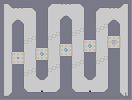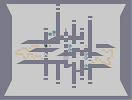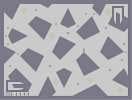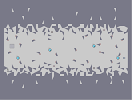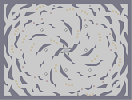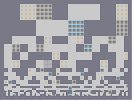stage 45: sidewinder stage 46: disurrected stage 47: golddust stage 48: gamma decay stage 49: call of the void stage 50: you're already dead

Pages: (0)

### greetings

now playing

The font is really cool. 5/5 because that must have taken a lot of time.
now I shall leave a sideways emoticon in the demo data box
Demo Data 0:3

### yes

does the author get a notification?

### @tiki

numa has been down for a good majority of the time since this was published

and map floating works when users rate maps, higher ratings mean they'll be higher on the list for a longer time
It's only been posted for 1.5 days, and NUMA's been down for a third of that time, so it might as well have been up for a day.

### Talk about the ultimate map post

Literally 40 maps for one post. I thought only 2 at a time on front page.... jkjk. But in all seriousness, what determines which maps stay on front page/stay near the top? Cuz this mappack thing has been at the top for multiple days, and it doesn't even have that many comments. Moderator controls or what?

### 5aving the GOD-DAMN art!

The pack looks really good.I would try to speedrun all.

### Gnarly

Newton would be proud of.

### Lovely pack

Some very hard maps. But also very simple and fun. I especially loved 'sidewinder' and 'melt and join' too.

### i have faves this map

so that i can play your maps anytime i want to :D

### I'm in love

with that font, damn

### i am nancy

and i approve of this